## 5.2.2 Halogen Gas for Silicon Etching

When etching silicon, HBr plasma is chosen due to its high selectability against SiO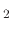, when compared to fluorocarbon based plasmas. When analyzing silicon etching under HBr plasma, the etch rate is assumed to only have the ion enhanced component, because the neutral component is negligible. Several experiments noted that under neutral-flux-only conditions no etching of silicon is observed with an HBr plasma , , . However, the LS framework can handle a model similar to the SF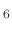silicon etching model suggested in  and implemented in the LS in  to be created for HBr/Osilicon etching. The corresponding balance equations can be written as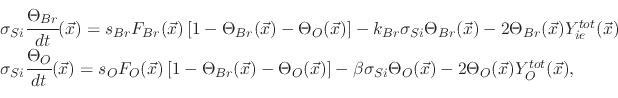(202)

where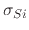is the surface density of silicon,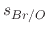is the sticking probability of bromine or oxygen on silicon,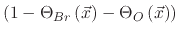is the fraction of free surface sites,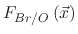are the total bromine or oxygen fluxes,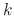is the bromine chemical etch rate constant,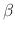is the oxygen chemical etch rate constant,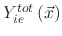is the total ion-enhanced etch rate,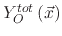is the total oxygen sputter rate, and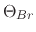and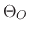refer to the total surface kinetic coverage of bromine and oxygen, respectively. The surface velocity is given by the total etch rate, as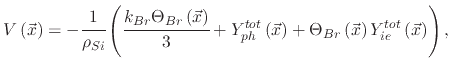(203)

whereis the silicon bulk density.

This model uses bromine properties from  in order to generate the topography of the etched silicon profile shown in Figure 5.8. The simulations use the following fluxes during the etching process: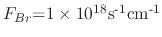,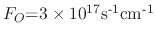, and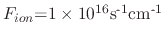.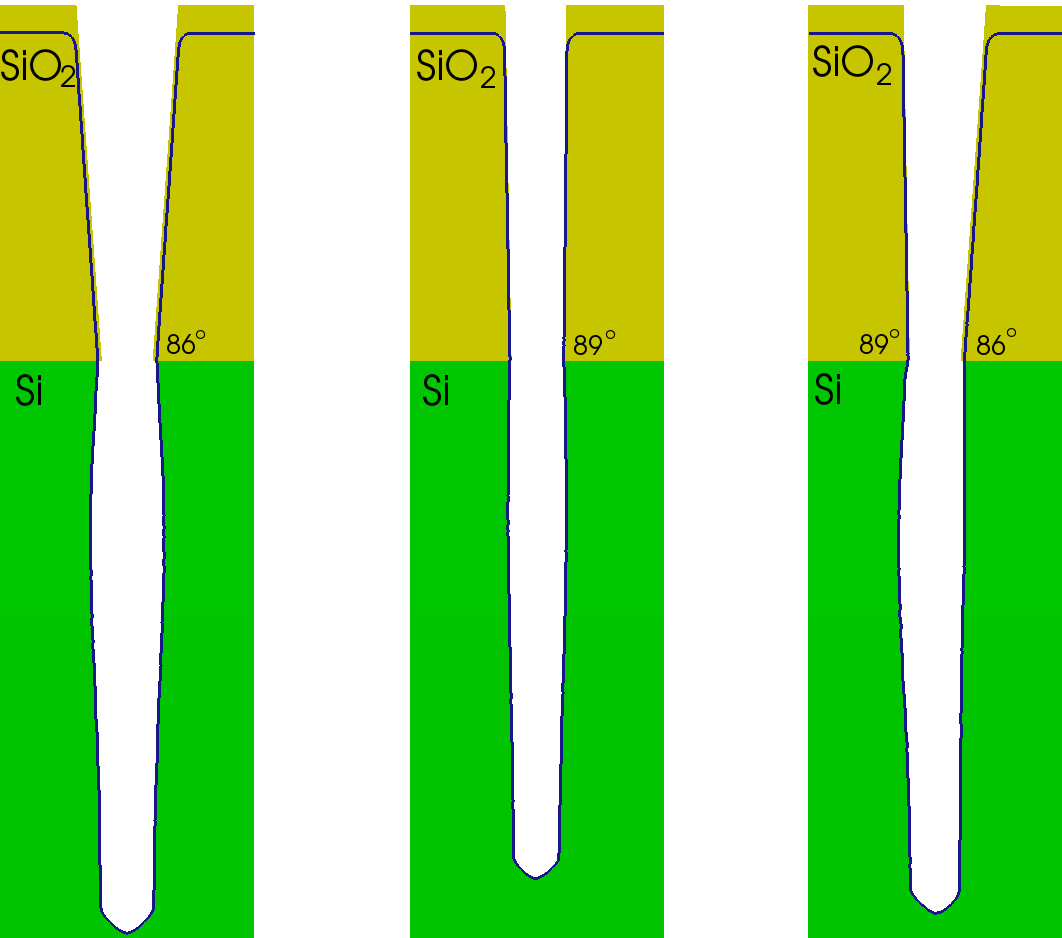The SiOmask opening is set to 70nm while etching continued for 410s, as was performed in . The simulation was performed in order to analyze the effects on the etching process, when the silicon dioxide layer has tapered walls. As can be seen, when the SiOis angled at 89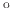, a relatively flat vertical sidewall is seen in the silicon. However, even a slight tapering, shown at 86, results in a silicon etched sidewall topography with an additional erosion width. These simulations confirm the experimental and simulated results from .

L. Filipovic: Topography Simulation of Novel Processing Techniques